Forecasting some probability vs. predicting values by some model (e.g., linear regression model).

Goal: Predicting some value on a scale.

The two parts to a model:

1. Defining a family of models that express a precise, but generic, pattern that you want to capture (e.g., as an equation that contains free parameters). For instance, linear models (that predict y from x) have the general form y = p1 + p2 * x and two parameters: an intercept p1 and a slope p2.

2. Generating a fitted model by finding the model from the family that is the closest to our data (assuming some distance measure).

Thus, the “best” model is not “true,” but the “closest” based on some criterion of distance.

Code from Chapter 23: Model basics of the r4ds book :

# setup:
library(tidyverse)
library(modelr)
library(ds4psy)
library(unikn)
options(na.action = na.warn)

# data:
dat <- modelr::sim1

Figure 19.1 shows a scatterplot of data: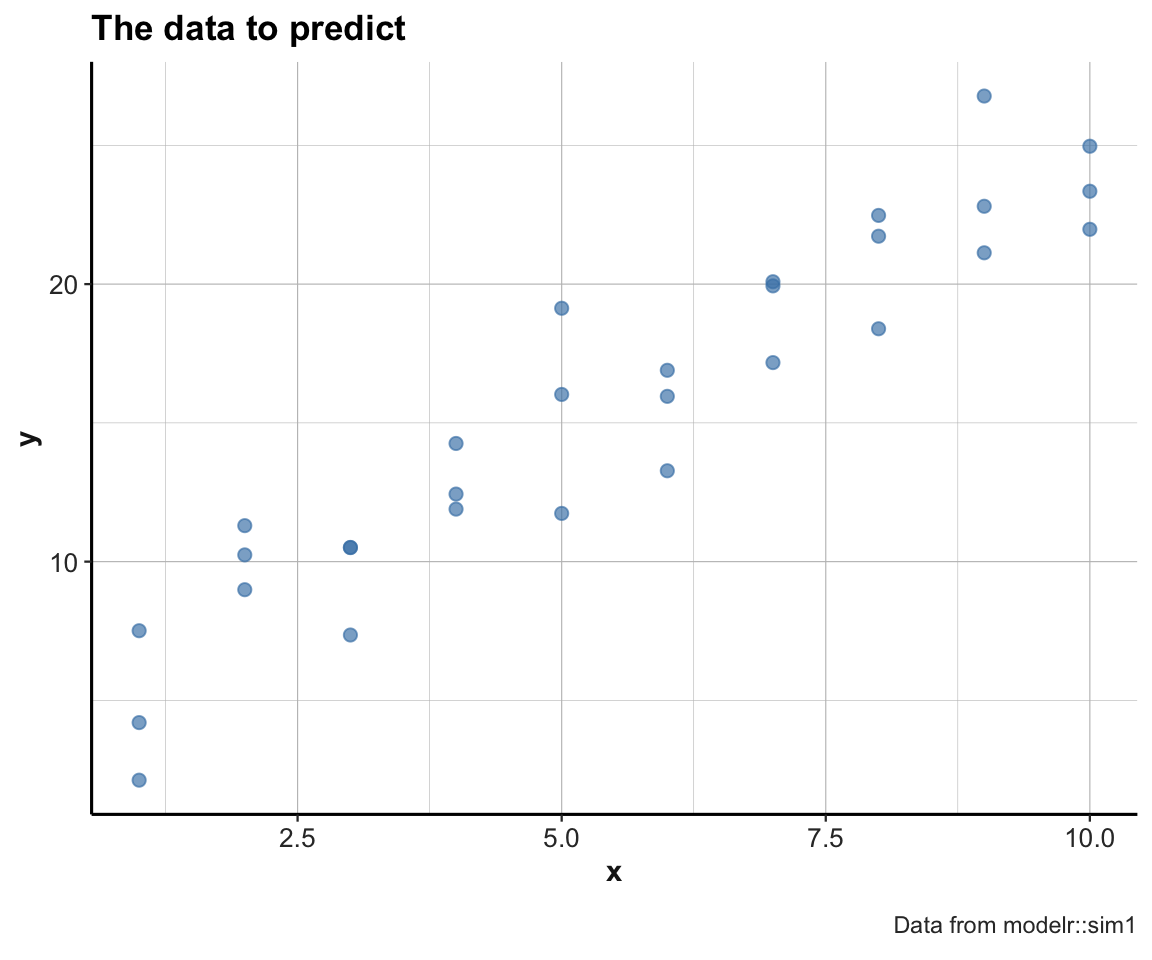Figure 19.1: A scatterplot of the to-be-predicted data.

#### Random models

Generate n linear models of form y = p1 + p2 * x:

n <- 100
set.seed(246)  # for reproducible randomness.

# Sample n random values for p1 and p2:
models <- tibble(
p1 = runif(n, -20, 20),
p2 = runif(n,  -5,  5)
)

ggplot(sim1, aes(x, y)) +
geom_abline(aes(intercept = p1, slope = p2), data = models, alpha = 1/5) +
geom_point(color = "steelblue", size = 2, alpha = 2/3) +
labs(title = "The data to predict and random linear models", caption = "Data from modelr::sim1") +
theme_ds4psy()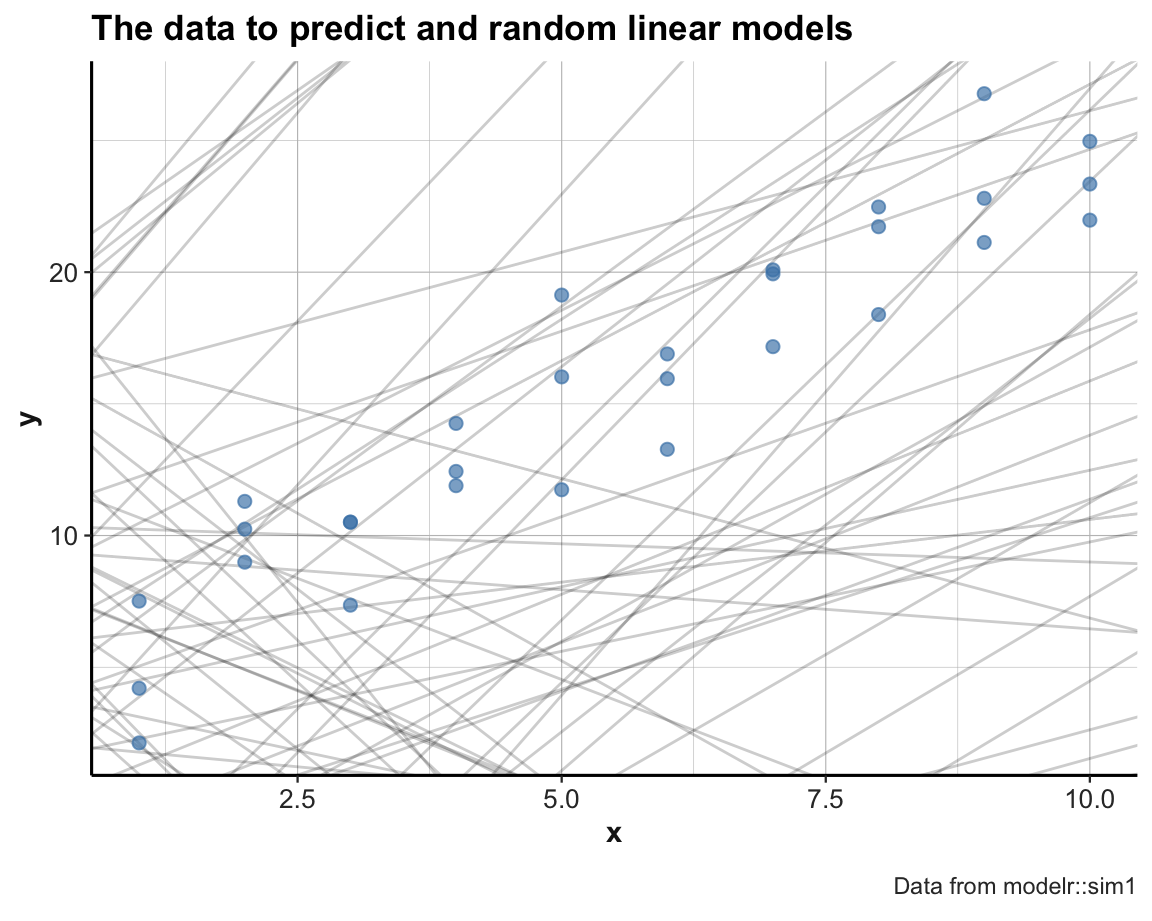Note usage of set.seed() for reproducible randomness.

Some random linear models appear to be ok, but most of them are pretty bad (i.e., do not describe the locations and relations of the data points very well).

We can improve the quality of our models by narrowing down the range of parameters from which we sample:

set.seed(123)  # for reproducible randomness.

# Sample n random values for p1 and p2:
models <- tibble(
p1 = runif(n, 0, 10),
p2 = runif(n, 0, 5)
)

ggplot(sim1, aes(x, y)) +
geom_abline(aes(intercept = p1, slope = p2), data = models, alpha = 1/5) +
geom_point(color = "steelblue", size = 2, alpha = 2/3) +
labs(title = "The data to predict and random linear models", caption = "Data from modelr::sim1") +
theme_ds4psy()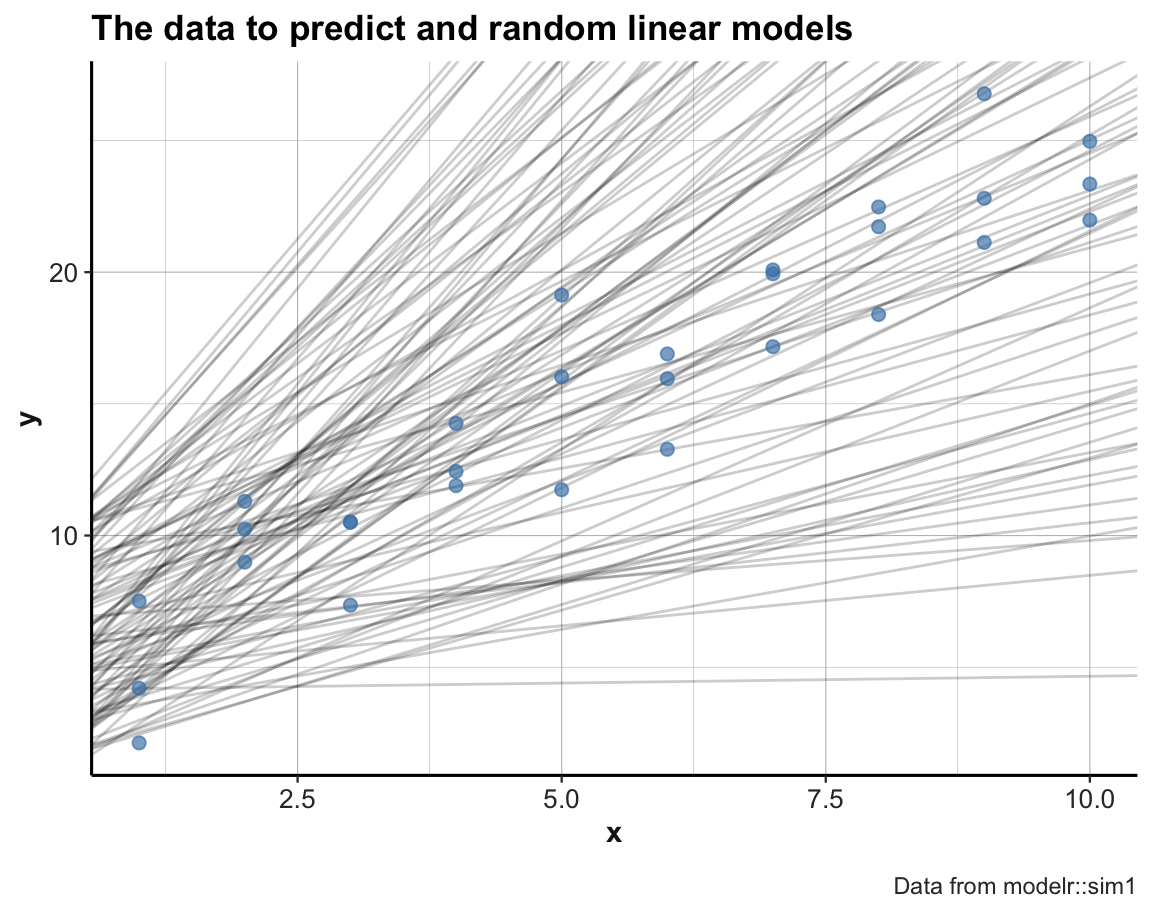The new set of models is much better. But to determine which is the best candidate among them, we need some way of comapring them to the data in a quantitative fashion.

To measure the performance of a model, we need to quantify the distance between the model and the actual data points.

A function that computes the predictions of a model (i.e., the model’s y values for some input data data$x), given the model parameters mp: # mp ... model parameters # Model predictions: model1 <- function(model, data) { model + model * data$x
}

# Generate predictions for various model parameters and dat:
model1(c(6, 3), dat)
#>    9  9  9 12 12 12 15 15 15 18 18 18 21 21 21 24 24 24 27 27 27 30 30 30 33
#>  33 33 36 36 36
model1(c(6, 2), dat)
#>    8  8  8 10 10 10 12 12 12 14 14 14 16 16 16 18 18 18 20 20 20 22 22 22 24
#>  24 24 26 26 26
model1(c(5, 3), dat)
#>    8  8  8 11 11 11 14 14 14 17 17 17 20 20 20 23 23 23 26 26 26 29 29 29 32
#>  32 32 35 35 35
model1(c(5, 2), dat)
#>    7  7  7  9  9  9 11 11 11 13 13 13 15 15 15 17 17 17 19 19 19 21 21 21 23
#>  23 23 25 25 25

Adding a distance function: root of the mean-squared deviation: The following function computes the difference between actual and predicted, square them, average them, and the take the square root:

distance <- function(model, data) {
diff <- data$y - model1(model, data) sqrt(mean(diff ^ 2)) } # Measure distance for models and dat: distance(c(6, 3), dat) #>  7.803283 distance(c(6, 2), dat) #>  2.60544 distance(c(5, 3), dat) #>  6.920964 distance(c(5, 2), dat) #>  2.190166 Alternative distance function (using 2 model parameters as two distinct arguments and only using the data of dat): dist <- function(p1, p2) { distance(c(p1, p2), data = dat) } # Checks: all.equal(dist(6, 3), distance(c(6, 3), dat)) #>  TRUE all.equal(dist(6, 2), distance(c(6, 2), dat)) #>  TRUE We can now compute the distance of each model in models: # using a for loop: md <- rep(NA, nrow(models)) for (i in 1:nrow(models)){ md[i] <- dist(models$p1[i], models$p2[i]) } md # Or directly: md_2 <- purrr::map2_dbl(models$p1, models$p2, dist) # Verify equality: all.equal(md, md_2) In one step: Compute the distance of all models (generated above) and arrange by lowest distance: models <- models %>% mutate(dist = purrr::map2_dbl(p1, p2, dist)) %>% arrange(dist) models # with a column for dist #> # A tibble: 100 x 3 #> p1 p2 dist #> <dbl> <dbl> <dbl> #> 1 4.15 2.05 2.13 #> 2 1.88 2.33 2.41 #> 3 2.32 2.20 2.43 #> 4 6.53 1.61 2.48 #> 5 5.51 2.05 2.50 #> 6 6.93 1.60 2.50 #> 7 6.56 1.85 2.52 #> 8 6.68 1.56 2.56 #> 9 3.80 1.86 2.64 #> 10 6.41 1.54 2.67 #> # … with 90 more rows We can now select the top_n models (i.e., those with the lowest overall distance to the sim1 data) and compare them to the data: # Plot the top models: top_n <- 10 # subset of models to plot: m2p <- models[1:top_n, ] # base R solution (given that models are sorted by -dist) m2p <- dplyr::filter(models, rank(dist) <= top_n) # dplyr solution # m2p pt <- paste0("The data to predict and the top ", top_n, " random linear models") ggplot(sim1, aes(x, y)) + geom_abline(aes(intercept = p1, slope = p2, color = dist), data = m2p, alpha = 4/5) + geom_point(color = "steelblue", size = 2, alpha = 2/3) + labs(title = pt, caption = "Data from modelr::sim1") + scale_color_gradient(low = "gold", high = Bordeaux) + theme_ds4psy()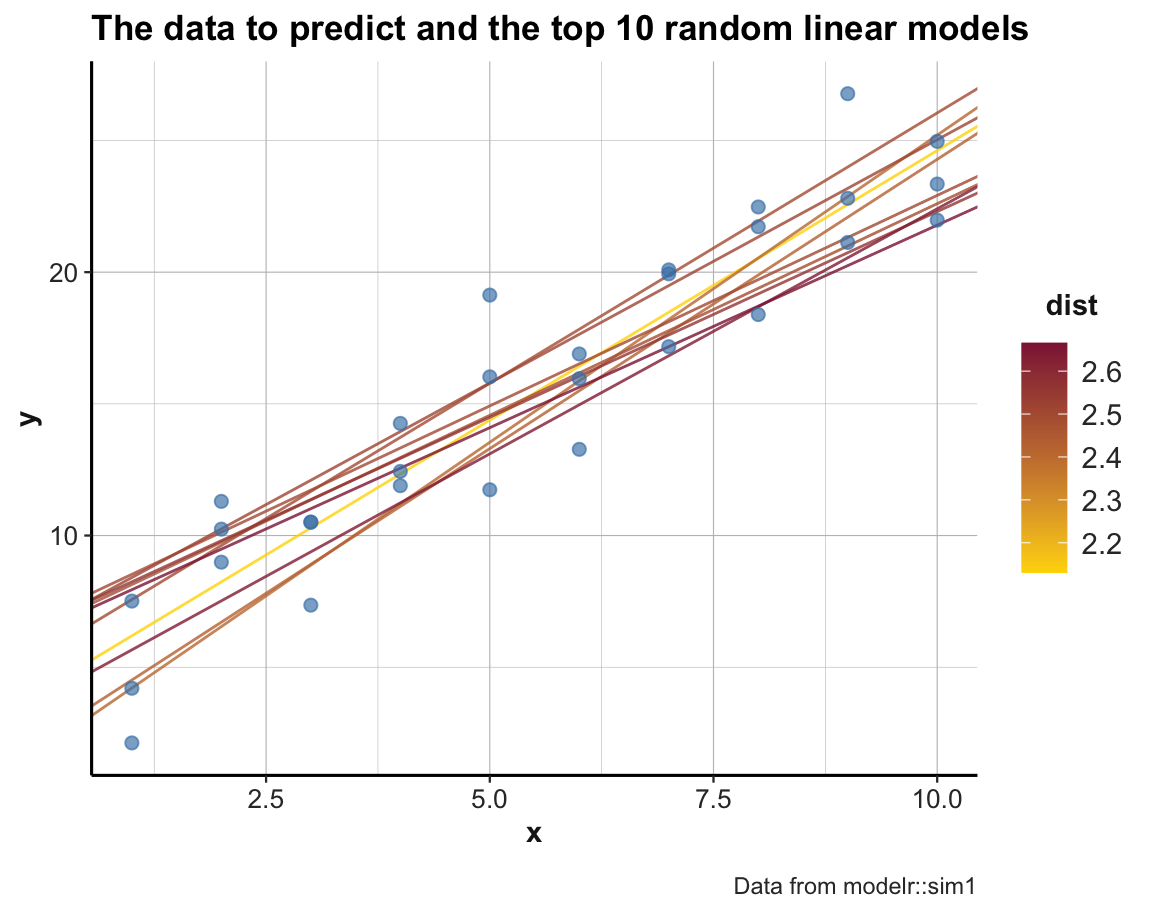An alternative way of looking at the models is by plotting the parameter pair p1 and p2 of each model as a scatterplot. To gain an impression about a model’s performance, we can color the corresponding point by -dist (i.e., models with a lower distance value will be colored in a lighter color). Additionally, the following command first draws the subset of the top_n models in a larger size and brighter color, effectively highlighting those models in the full scatterplot: ggplot(models, aes(p1, p2)) + geom_point(data = filter(models, rank(dist) <= top_n), size = 4, color = Seegruen) + geom_point(aes(color = dist), size = 2) + scale_color_gradient(low = "gold", high = Bordeaux) + theme_ds4psy()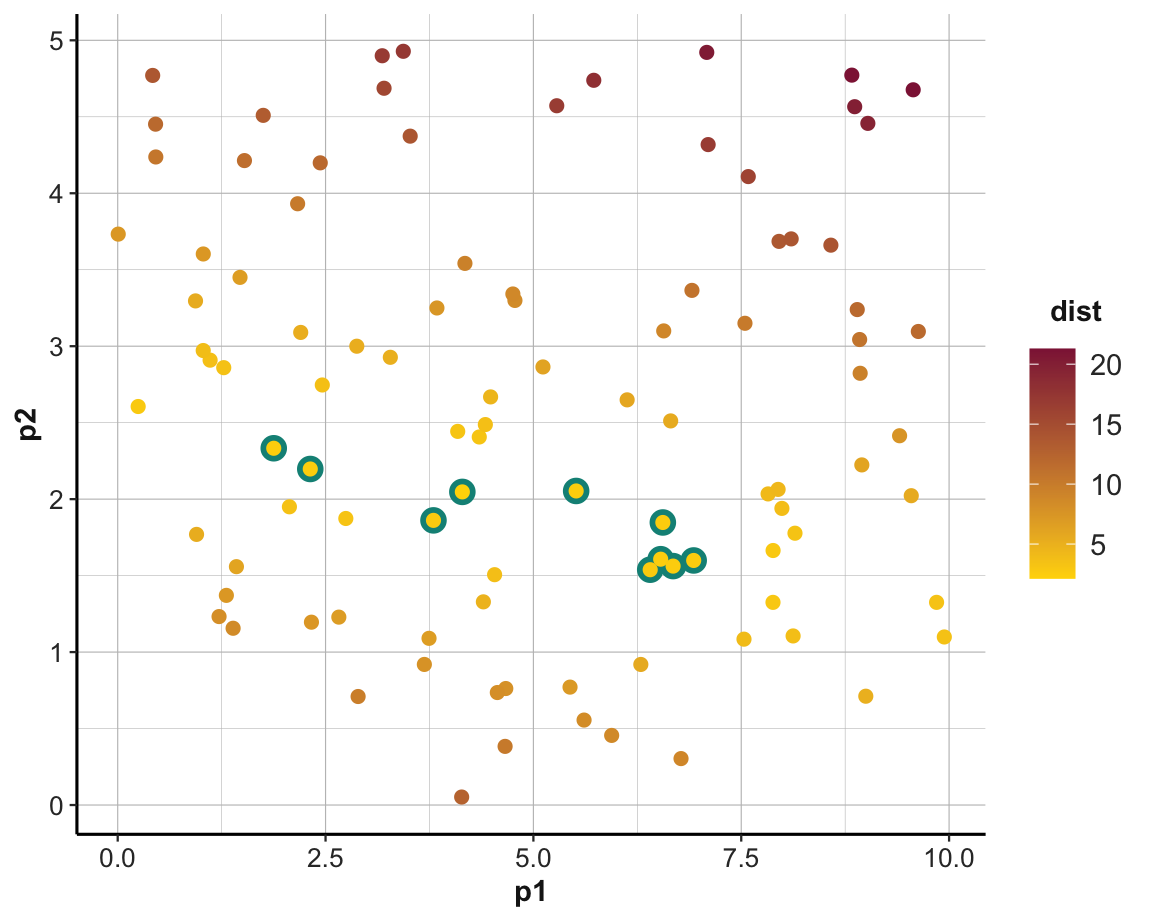#### Minimizing distance An important part of quantitative modeling is to find parameters that would optimize (i.e., maximize or minimize) some measure. There are multiple ways of doing this. If we are considering a one-dimensional range of values, the optimize() function (of the stats package) searches this range (from some lower to some upper value) for a minimum (or maximum) of the first argument of some function f: curve(expr = (x - 1/3)^2, from = -1, to = 2, col = "deepskyblue", lwd = 2, ylab = "y") # As a function: fn <- function(x, a) {(x - a)^2} # x as 1st parameter (to be optimized) (mn <- optimize(fn, c(-1, 2), a = 1/3)) #>$minimum
#>  0.3333333
#>
#> $objective #>  0 (mx <- optimize(fn, c(-1, 2), a = 1/3, maximum = TRUE)) #>$maximum
#>  1.999924
#>
#> $objective #>  2.777525 (mx_2 <- optimize(fn, c(-1, 1), a = 1/3, maximum = TRUE)) # Note limited range #>$maximum
#>  -0.999959
#>
#> $objective #>  1.777668 # Not as lists: # (mx <- unlist(mx)) # as named vector points(x = mn$minimum, y = mn$objective, col = "olivedrab3", pch = 16, cex = 2) # using mn points(x = mx$maximum, y = mx$objective, col = "brown2", pch = 17, cex = 2) # using mx points(x = mx_2$maximum, y = mx_2$objective, col = "steelblue", pch = 18, cex = 2) # using mx_2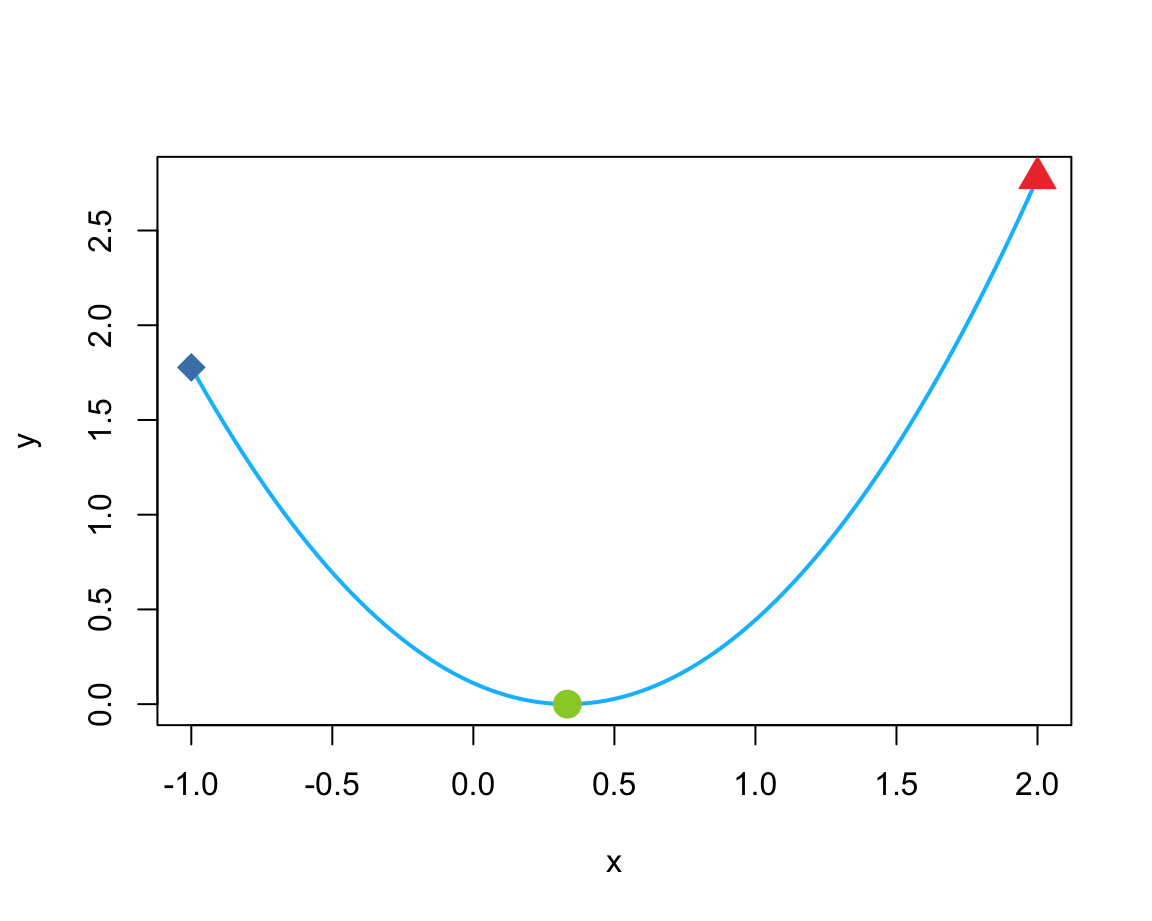Examples based on the documentation of optimize(): # Example 2: fn <- function(x) {x^3 * (x - 1)} plot(fn, col = "deepskyblue", lwd = 2) # Minimum: (mn <- optimize(fn, lower = 0, upper = 2)) #>$minimum
#>  0.7500078
#>
#> $objective #>  -0.1054687 points(x = mn$minimum, y = mn$objective, col = "olivedrab3", pch = 16, cex = 2) # using mx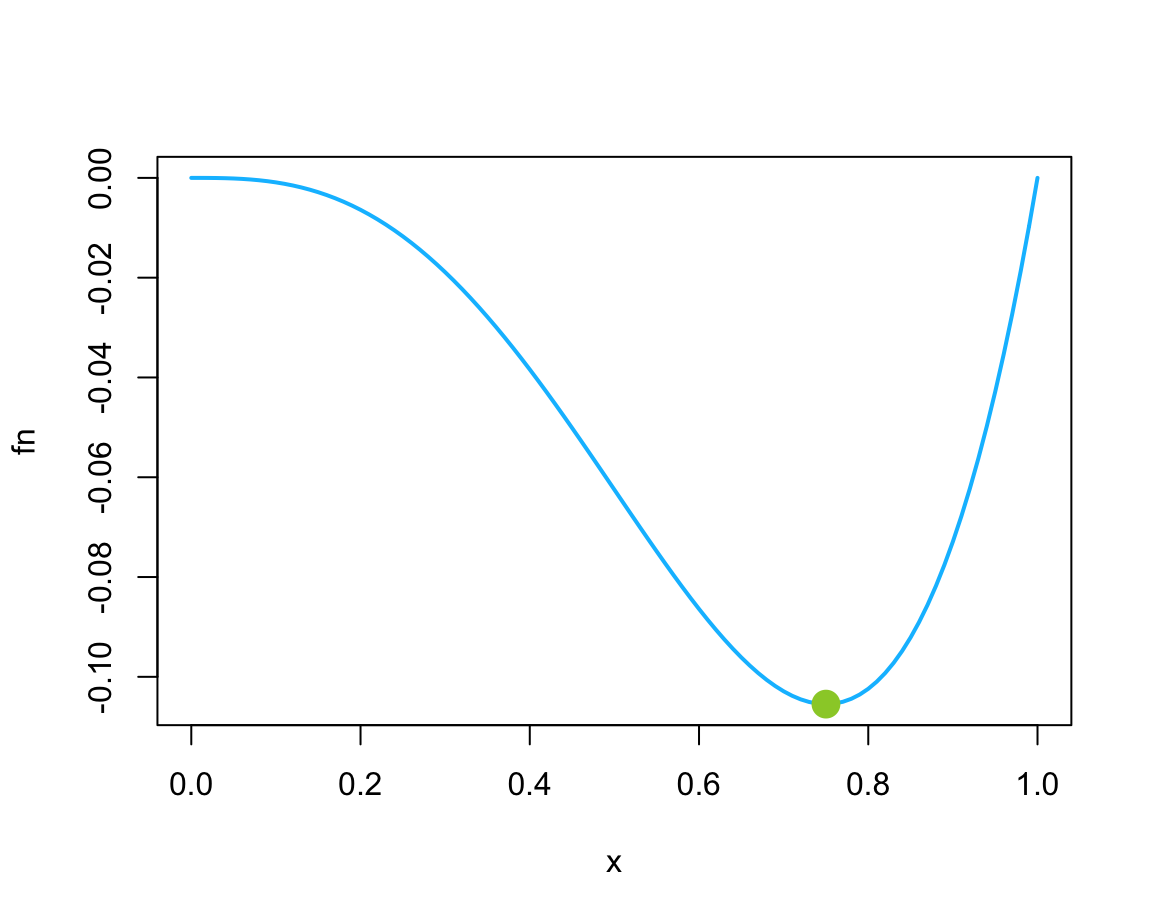# See where a function is evaluated: optimize(function(x) x^3 * (print(x) - 1), lower = 0, upper = 2) #>  0.763932 #>  1.236068 #>  0.472136 #>  0.6666667 #>  0.7777988 #>  0.7471242 #>  0.7502268 #>  0.7499671 #>  0.7500078 #>  0.7500485 #>  0.7500078 #>$minimum
#>  0.7500078
#>
#> $objective #>  -0.1054687 # Warning: # A "wrong" solution with an unlucky interval and piecewise constant f(): f <- function(x) ifelse(x > -1, ifelse(x < 4, exp(-1/abs(x - 1)), 10), 10) fp <- function(x) { print(x); f(x) } plot(f, -2,5, ylim = 0:1, col = "red")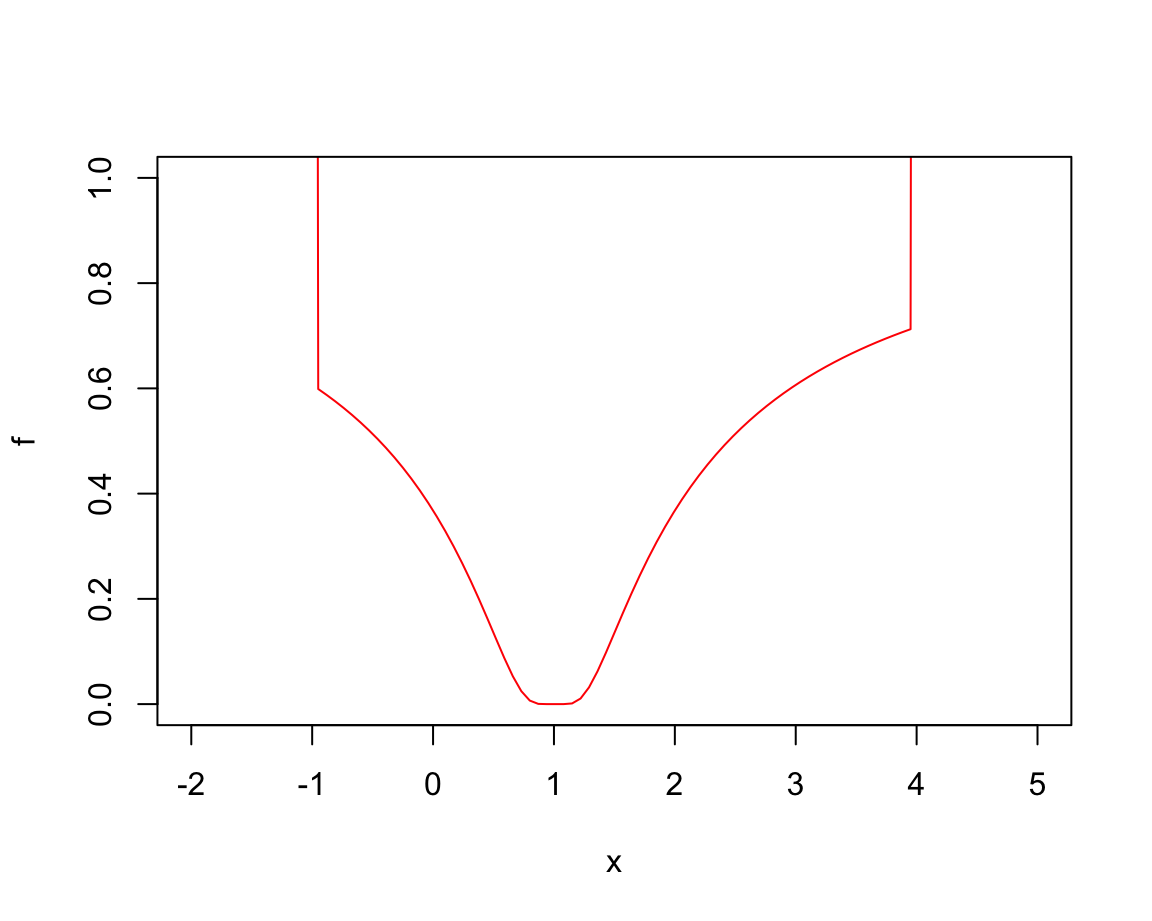optimize(fp, c(-4, 20)) # fails to find the minimum #>  5.167184 #>  10.83282 #>  14.33437 #>  16.49845 #>  17.83592 #>  18.66253 #>  19.1734 #>  19.48913 #>  19.68427 #>  19.80487 #>  19.8794 #>  19.92547 #>  19.95393 #>  19.97153 #>  19.9824 #>  19.98913 #>  19.99328 #>  19.99585 #>  19.99743 #>  19.99841 #>  19.99902 #>  19.99939 #>  19.99963 #>  19.99977 #>  19.99986 #>  19.99991 #>  19.99995 #>  19.99995 #>$minimum
#>  19.99995
#>
#> $objective #>  10 optimize(fp, c(-7, 20)) # finds minimum #>  3.313082 #>  9.686918 #>  -0.6261646 #>  1.244956 #>  1.250965 #>  0.771827 #>  0.2378417 #>  1.000451 #>  0.9906964 #>  0.9955736 #>  0.9980122 #>  0.9992315 #>  0.9998411 #>  0.9996083 #>  0.9994644 #>  0.9993754 #>  0.9993204 #>  0.9992797 #>  0.9992797 #>$minimum
#>  0.9992797
#>
#> $objective #>  0 optimize(fp, c(-2, 5)) #>  0.6737621 #>  2.326238 #>  -0.3475242 #>  0.9935203 #>  1.075221 #>  1.034342 #>  1.013931 #>  1.003726 #>  0.998623 #>  1.001174 #>  0.9998987 #>  1.000538 #>  1.000294 #>  1.000143 #>  1.00005 #>  0.999992 #>  0.9999513 #>  0.9999513 #>$minimum
#>  0.9999513
#>
#> $objective #>  0 Here, we explore two different methods (both from the stats package that comes with R): General intuition: Given the scatterplot of our data (shown in Figure 19.1), we have a pretty good idea that we are looking for a linear model. 1. General-purpose optimization (using optim()) 2. Fitting a linear model (using lm()) • ad 1: Using optim() to determine the best parameter pair: Intuition: Given a fn = distance and data, optim() will use an algorithm to find the parameter combination par that minimize the value of distance. # sim1 best <- optim(par = c(0, 0), fn = distance, data = sim1) best$par # vector of the 2 best parameters (i.e., minimizing fn for data)
#>  4.222248 2.051204

ggplot(sim1, aes(x, y)) +
geom_point(color = "steelblue", size = 2, alpha = 2/3) +
geom_abline(intercept = best$par, slope = best$par, color = "gold") +
labs(title = "The best linear model to predict the data",
caption = "Data from modelr::sim1") +
theme_ds4psy()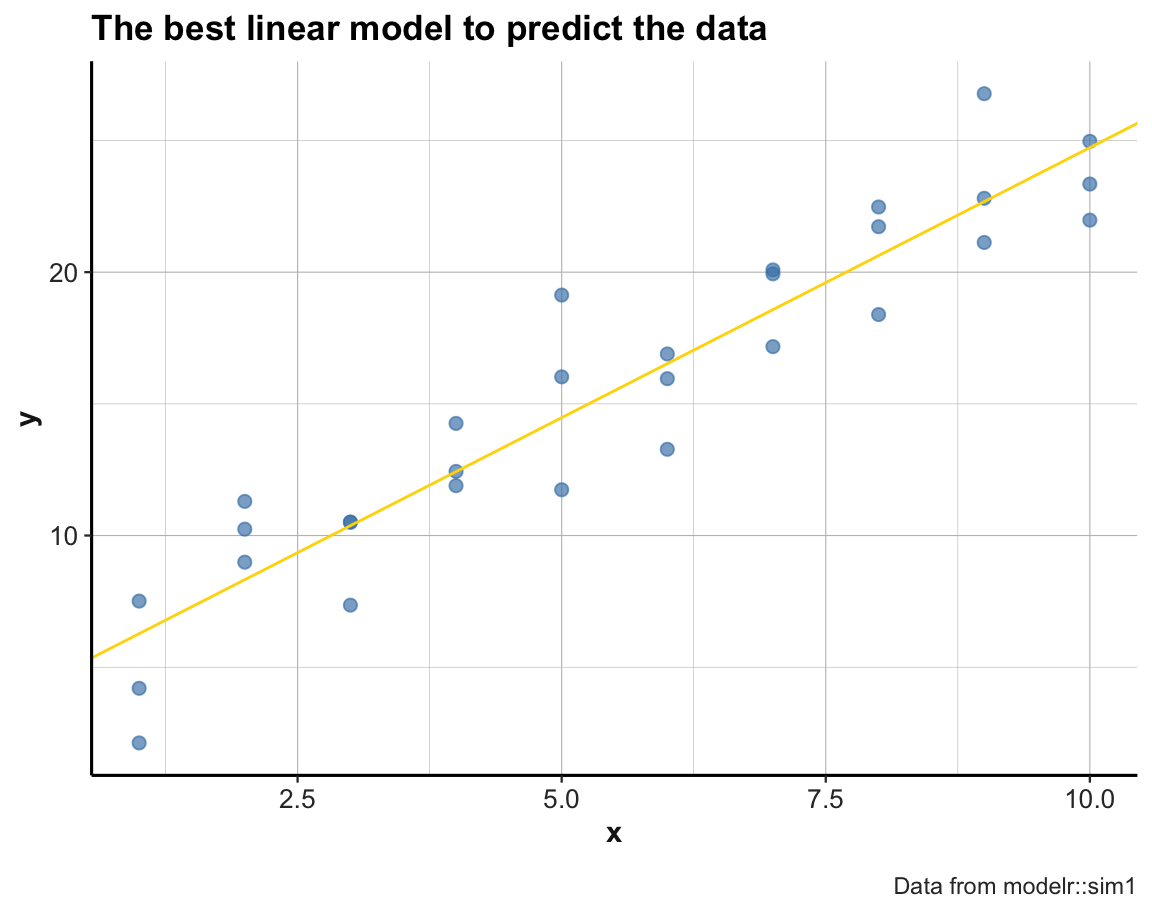• ad 2: Using lm() for fitting a linear model:
sim1_mod <- lm(y ~ x, data = sim1)
coef(sim1_mod)
#> (Intercept)           x
#>    4.220822    2.051533

For fitting other datasets and types of functions, using glm() or nlm() may be indicated.

+++ here now +++

### 19.4.1 ToDo 2

Generalize and use a more meaningful dataset:

• Candidate data: father.son or galton (both from the UsingR package)

• analysis

### References

Wickham, H., & Grolemund, G. (2017). R for data science: Import, tidy, transform, visualize, and model data. O’Reilly Media, Inc. http://r4ds.had.co.nz